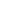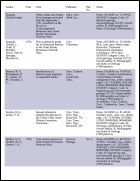Large Table ExampleThis example shows how to draw a multi-page table. ABCpdf does not provide table drawing routines itself so this example uses a Table Class to position the table elements.Setup

 We start by creating our document object and reading the data for our table. For the purposes of this example, we'll assume that our data is in a standard tab delimited format. '... read the text Set fso = CreateObject("Scripting.FileSystemObject") Set f = fso.OpenTextFile(Server.MapPath("text7.txt"), 1) theText = f.ReadAll() Set theDoc = Server.CreateObject("ABCpdf12.Doc") ' set up document theDoc.FontSize = 12 theDoc.Rect.Inset 20, 20Rotate

 We create a new table object and focus on our document. Focusing tells the table what rectangle it can occupy (it takes the current document rectangle) and how many columns of data it should be prepared for. Columns are assigned relative widths and expand horizontally to fit the table rectangle. Here, we're specifying six columns and a number of relative widths. Columns one and four are not assigned a width so they default to a relative width of one. Finally, we're padding the cells so there are gaps between the rows and columns. Set theTable = New Table ' focus the table on the document theTable.Focus theDoc, 6 ' some columns extra width theTable.Width(0) = 2 theTable.Width(2) = 3 theTable.Width(3) = 2 theTable.Width(5) = 4 ' ensure columns are padded theTable.Padding = 5Results

Using a large quantity of input data, we get the following output.table2.pdf [Page 1]table2.pdf [Page 2]table2.pdf [Page 3]table2.pdf [Page 4]table2.pdf [Page 5]